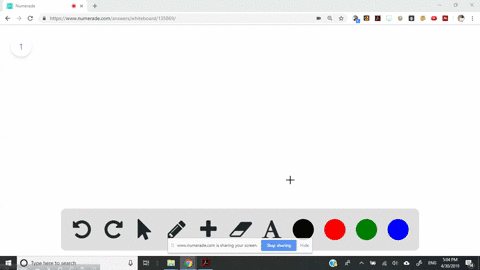Sign up for our free STEM online summer camps starting June 1st!View Summer Courses### The following diagram represents the collection o…

04:08University of New Mexico

Need more help? Fill out this quick form to get professional live tutoring.

Get live tutoring
Problem 3

The following diagram represents the collection of elements formed by a decomposition reaction. (a) If the blue spheres represent $N$ atoms and the red ones represent $\mathrm{O}$ atoms, what was the empirical formula of the original compound? (b) Could you draw a diagram representing the molecules of the compound that had been decomposed? Why or why not? [Section 3.2$]$

a) $\mathrm{NO}_{2}$
b) the simplest ratio

## Discussion

You must be signed in to discuss.

## Video Transcript

for Option A a compound off in on dhe oh decomposes in such a way that the number off oxygen to molecule is doubled the number off and two molecule, which means that the empirical formula must be in 02 for option B. We cannot draw a diagram representing the molecule off the compound, since we don't know whether it's molecular formula is same as the empirical formula. And auto represents the simplest racial between nitrogen on oxygen. So, Dan, sorry for B is no.